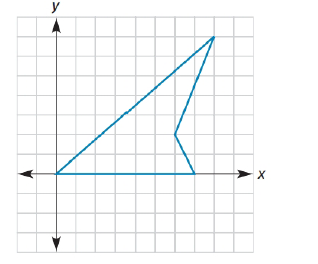Chapter 10.4, Problem 33EElementary Geometry For College St...

7th Edition
Alexander + 2 others
ISBN: 9781337614085

Solutions

Chapter
SectionElementary Geometry For College St...

7th Edition
Alexander + 2 others
ISBN: 9781337614085
Textbook Problem

Would the theorem of Exercise 7 remain true for a concave quadrilateral like the one shown?Exercise 33.

To determine

The analytic proof for the given theorem “Would the theorem of Exercise 7 remain true for the given concave quadrilateral”

Explanation

Given figure is,

The above figure shows the quadrilateral.

And the theorem of Exercise 7 is “The line segments that join the midpoints of the consecutive sides of a quadrilateral form a parallelogram”

To prove “The line segments that join the midpoints of the consecutive sides of a quadrilateral form a parallelogram” for the given figure, follow as below,

The coordinates of the quadrilateral ABCD is as shown in the figure,

Let R, S, T, V are the midpoint of AB, BC, CD and AD.

Joining the R, T, TV and VR as shown in the figure.

Midpoint of AB = S = 7+02, 0+02

Midpoint of AB = S = 72,02

Midpoint of AB = S = 3.5, 0

Midpoint of BC = T = 7+62, 0+22

Midpoint of BC = T = 132,22

Midpoint of BC = T = 6.5, 1

Midpoint of CD = V = 8+62, 7+22

Midpoint of CD = V = 142,92

Midpoint of CD = V = 7, 4.5

Midpoint of AD = R = 8+02, 7+02

Midpoint of AD = R = 82,72

Midpoint of AD = R = 4, 3.5

Determining the slopes as below,

mST-=1-06

Still sussing out bartleby?

Check out a sample textbook solution.

See a sample solution

The Solution to Your Study Problems

Bartleby provides explanations to thousands of textbook problems written by our experts, many with advanced degrees!

Get Started

Find more solutions based on key concepts